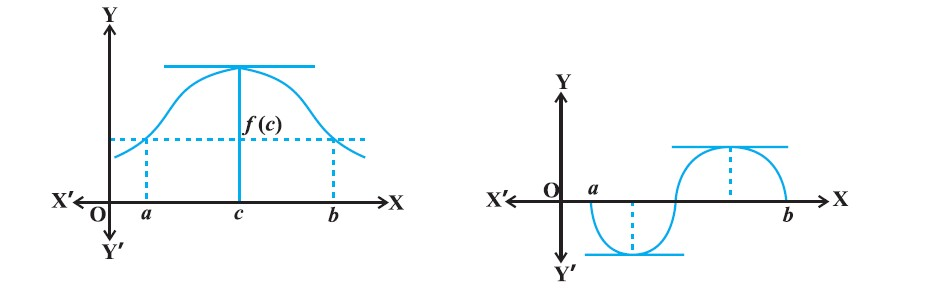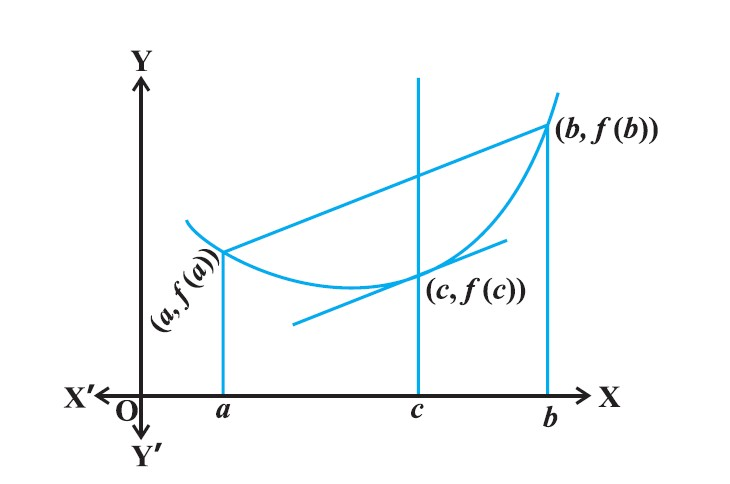Mathematics Rolles Theorem and Lagrange Mean Value Theorem For CBSE-NCERT
Click for Only Video

### Topic covred

star Rolle’s Theorem
star Mean Value Theorem

### Rolle’s Theorem :\color{green} ✍️ Let f : [a, b] → R be continuous on  [a, b] and differentiable on (a, b),
such that color{red}{f(a) = f(b)}, where a and b are some real numbers.

\color{green} ✍️ Then there exists some c in (a, b) such that color{blue}{f ′(c) = 0 }.

\color{green} ✍️ In Fig, In each of the graphs, the slope becomes zero at least at one point.
That is precisely the claim of the Rolle’s theorem.
Q 3155434364Verify Rolle’s theorem for the function y = x^2 + 2, a = – 2 and b = 2 .
Class 12 Chapter 5 Example 42Solution:

The function y = x^2 + 2 is continuous in [– 2, 2] and differentiable in (– 2, 2).
Also f (– 2) = f ( 2) = 6 and hence the value of f (x) at – 2 and 2 coincide. Rolle’s
theorem states that there is a point c ∈ (– 2, 2), where f′ (c) = 0. Since f′ (x) = 2x, we
get c = 0. Thus at c = 0, we have f′ (c) = 0 and c = 0 ∈ (– 2, 2).

### Mean Value Theorem :\color{green} ✍️ Let f : [a, b] → R be a continuous function on [a, b] and differentiable on (a, b).

\color{green} ✍️ Then there exists some c in (a, b) such that

color{red}{f'(c) =(f(b)-f(a))/(b-a)}

=> Mean Value Theorem (MVT) is an extension of Rolle’s theorem.

=> In fig y = f(x) is given, We've already interpreted f ′(c) as the slope of the tangent to the curve y = f (x) at (c, f (c)).

\color{green} ✍️ From the Fig color{blue}{((f(b)-f(a))/(b-a))} is the slope of the secant drawn between (a, f (a)) and (b, f (b)).

=> color{blue}{"The MVT states that there is a point" \ \ c \ \ "in" (a, b) "such that the slope of the tangent at"}
color{blue}{ (c, f(c)) "is same as the slope of the secant between" (a, f (a)) "and" (b, f (b))}.

\color{green} ✍️ In other words, there is a point c in (a, b) such that the tangent at (c, f (c)) is parallel to the secant between (a, f (a)) and (b, f (b)).
Q 3175434366Verify Mean Value Theorem for the function f (x) = x^2 in the interval [2, 4].
Class 12 Chapter 5 Example 43Solution:

The function f (x) = x^2 is continuous in [2, 4] and differentiable in (2, 4) as its
derivative f′ (x) = 2x is defined in (2, 4).

Now, f (2) = 4 and f (4) = 16. Hence

 ( f(b) - f(a) )/(b-a) = (16-4)/(4-2) = 6

MVT states that there is a point c ∈ (2, 4) such that f′ (c) = 6. But f′ (x) = 2x which
implies c = 3. Thus at c = 3 ∈ (2, 4), we have f′ (c) = 6.